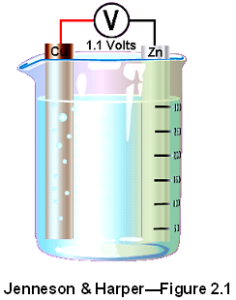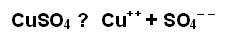Home / Electrical MCQs / Electrochemistry MCQs with Answers

Want create site? Find Free WordPress Themes and plugins.
1. In the electroplating process an external voltage is applied across a pair of electrodes, causing:

 A. a current to flow through an electrolyte
 B. an electroplate to form on an electrolyte
 C. an alternating voltage in a magnetic chemical
 D. a chemical to produce a negative voltage on a positive electrode

2. In the electroplating process the electrode connected to the positive polarity of the supply is called the anode and the electrode connected to the negative is:

 A. also called the anode
 B. called the cathode
 C. called the electrolyte
 D. called the electrolysis

3. Primary cells are electrochemical devices that convert chemical energy into:

 A. mechanical energy
 B. electrostatic energy
 C. electric energy
 D. heat energy

4. Secondary cell chemistries permit the cell to be recharged by:

 A. reversing the polarity of the discharged cell
 B. alternating the electrodes with a primary cell of the same type
 C. replacing the chemicals in the electrolyte
 D. reversing the current flow using another energy source

5. Look at the following figure:The diagram shows a simple voltaic cell consisting of two electrodes, one of copper and the other of zinc, immersed in a solution of dilute hydrochloric acid. In this cell the copper electrode is:

 A. positive and the zinc electrode is negative
 B. negative and the zinc electrode is positive
 C. positive and the electrolyte is positive
 D. negative and the zinc electrode is negative

6. This simple cell is not very practical, because the copper electrode becomes covered with hydrogen gas, preventing hydrogen ions from taking further electrons from the surface. This effect is known as:

 A. neutralization
 B. polarization
 C. local action
 D. hydrogenation

7. To reduce the local action in a simple cell it is necessary to:

 A. replace the zinc electrodes with copper
 B. increase the polarization effect in the electrolyte
 C. use zinc electrodes that are as pure as possible
 D. circulate currents between the impurities and the electrode

8. Two dissimilar metals in an electrolyte form a primary cell. The voltage across their terminals depends on the:

 A. acidic nature of the electrolyte used
 B. physical size of the electrodes
 C. positive ions available at the negative terminal
 D. electrode potential of the metals used

9.
A simple voltaic cell has electrodes of copper and aluminum. The EMF of the cell will be:

 A. 2.01 V
 B. 1.56 V
 C. 1.33 V
 D. 1.10 V

10. If a small amount of an acid, alkali or salt is added to water, it can become:

 A. a very good insulator
 B. quite a good conductor
 C. a semi-conducting diode
 D. an electro-positive acceptor

11. If an electric current flows through the electrolyte, it dissociates the molecules of the substances in solution into:

 A. protons
 B. neutrons
 C. ions
 D. atoms

12. Look at the following diagram:

The diagram shows two copper plates that are immersed in a solution of water and copper sulphate. A voltage has been applied to the two electrodes, and a current is flowing. This current will cause the, copper sulphate to break down into:

 A. negative copper ions and negative sulphate ions
 B. negative copper ions and positive sulphate ions
 C. positive copper ions and positive sulphate ions
 D. positive copper ions and negative sulphate ions

13. 13.The following equation shows the shows the action if copper sulphate is dissolved in water and a current passed through it.The arrow pointing to the right indicates dissociation, while the arrow pointing to the left indicates that:

 A. recombination can take place to form neutral molecules of copper sulphate
 B. the negative ions in the solution have a deficiency of electrons
 C. copper can be split from the SO4 sulphate radical in the electrode
 D. copper sulphate molecules will always return a positive charge to the electrode

14. When an electric current is passed through an electrolyte:

 A. gasses cannot be released
 B. gases may be released
 C. the electrodes will sink to the bottom of the electrolyte
 D. the electrolyte will convert to liquid form for later use

15. When the electrolysis method for transferring metal from one electrode to another is used on other metal components to place a metal coating on the surface of that metal, the b process is called:

 A. crystallization
 B. electro-current normalization
 C. electroplating
 D. electromotive tempering

16.
The drawing shows the basic requirements of an electroplating bath. In the drawing, the:

 A. copper electrode will be zinc plated
 B. zinc spoon will go into solution in the electrolyte
 C. copper electrode will be plated with sulphate
 D. zinc spoon will be copper plated

17. Anodizing creates an oxide layer on the surface of a metal that is:

 A. thicker than the naturally occurring oxide
 B. thinner than the naturally occurring oxide
 C. much lighter than the naturally occurring oxide
 D. a more porous surface than the naturally occurring oxide

18. The Electroerosion process can be used to:

 A. generate an EMF of erosion
 B. remove metal as a means of manufacturing parts
 C. force an electrolyte through a conductor
 D. electroplate high-reliability connections with gold

19. Faraday’s first law of electrochemical deposition states that the mass of metal deposited is directly proportional to the:

 A. voltage applied to the electrodes
 B. amount of electrolyte in the electroplating tank
 C. the current and the duration of its flow
 D. power applied to the electrolyte flowing

20. Faraday’s second law of electrochemical deposition states that the mass of metal deposited by a quantity of electric charge is:

 A. inversely proportional to the amount of voltage applied to the electrolyte
 B. proportional to the electrolytic refining constant for the electrolyte
 C. inversely proportional to the electrochemical equivalent of the metal
 D. proportional to the electrochemical equivalent of the metal

21. The following formula can be used to determine the amount of metal deposited by an electroplating bath.In the formula, ‘z’ stands for the:

 A. electrochemical equivalent of the metal
 B. current flowing in amperes
 C. mass of metal deposited in coulombs
 D. time of current flow in seconds

22.
An electrolytic refining bath has a constant current of 4500 A. The amount of zinc deposited by this current in 18 hours will be:

 A. 81.0 kg
 B. 98.85 kg
 C. 131.8 kg
 D. 146.5 kg

23. Processes using the electrolytic method of metal deposition:

 A. do not produce metals with a high purity
 B. usually operates above 95% efficiency
 C. are expensive and require large amounts of electrical energy
 D. are fairly inexpensive with energy costs

24.
The diagram shows the connection of a copper conductor to an aluminum conductor. The copper and aluminum will act as electrodes when an electrolyte is present from condensation or rain. In this situation, the metal with the higher potential:

 A. becomes the cathode of a simple cell
 B. become zinc plated from the fastener
 C. is the copper busbar
 D. will corrode away

25. Dissimilar metals in damp ground and adjacent to each other may produce stray currents. These stray currents can cause underground pipes and cables to:

 A. become corroded by electrolytic action
 B. generate very high voltages
 C. last longer between maintenance inspections
 D. generate static a c. voltages

26. Electrolytic corrosion of bronze propellers on ships can be minimized by bolting blocks of zinc to the metal hulls adjacent to the propellers. In this situation, the blocks are called:

 A. sacrificial cathodes
 B. sacrificial anodes
 C. catalytic converters
 D. electrolytic generators

27. When using the Cathodic protection method of electrolytic protection, an external DC voltage is applied between the equipment to be protected and:

 A. the supply active
 B. a c. supply voltage
 C. the surrounding earth
 D. the equipment

Did you find apk for android? You can find new Free Android Games and apps.